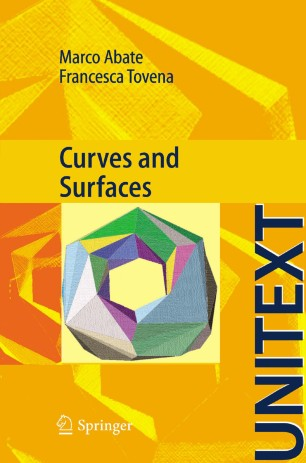# Curves and Surfaces

• Marco Abate
• Francesca TovenaTextbook

Part of the UNITEXT book series (UNITEXT)

Also part of the La Matematica per il 3+2 book sub series (UNITEXTMAT)

1. Front Matter
Pages I-XIII
2. Marco Abate, Francesca Tovena
Pages 1-65
3. Marco Abate, Francesca Tovena
Pages 67-116
4. Marco Abate, Francesca Tovena
Pages 117-164
5. Marco Abate, Francesca Tovena
Pages 165-246
6. Marco Abate, Francesca Tovena
Pages 247-301
7. Marco Abate, Francesca Tovena
Pages 303-344
8. Marco Abate, Francesca Tovena
Pages 345-380
9. Back Matter
Pages 381-396

### Introduction

The book provides an introduction to Differential Geometry of Curves and Surfaces. The theory of curves starts with a discussion of possible definitions of the concept of curve, proving in particular the classification of 1-dimensional manifolds. We then present the classical local theory of parametrized plane and space curves (curves in n-dimensional space are discussed in the complementary material): curvature, torsion, Frenet’s formulas and the fundamental theorem of the local theory of curves. Then, after a self-contained presentation of degree theory for continuous self-maps of the circumference, we study the global theory of plane curves, introducing winding and rotation numbers, and proving the Jordan curve theorem for curves of class C2, and Hopf theorem on the rotation number of closed simple curves. The local theory of surfaces begins with a comparison of the concept of parametrized (i.e., immersed) surface with the concept of regular (i.e., embedded) surface. We then develop the basic differential geometry of surfaces in R3: definitions, examples, differentiable maps and functions, tangent vectors (presented both as vectors tangent to curves in the surface and as derivations on germs of differentiable functions; we shall consistently use both approaches in the whole book) and orientation. Next we study the several notions of curvature on a surface, stressing both the geometrical meaning of the objects introduced and the algebraic/analytical methods needed to study them via the Gauss map, up to the proof of Gauss’ Teorema Egregium. Then we introduce vector fields on a surface (flow, first integrals, integral curves) and geodesics (definition, basic properties, geodesic curvature, and, in the complementary material, a full proof of minimizing properties of geodesics and of the Hopf-Rinow theorem for surfaces). Then we shall present a proof of the celebrated Gauss-Bonnet theorem, both in its local and in its global form, using basic properties (fully proved in the complementary material) of triangulations of surfaces. As an application, we shall prove the Poincaré-Hopf theorem on zeroes of vector fields. Finally, the last chapter will be devoted to several important results on the global theory of surfaces, like for instance the characterization of surfaces with constant Gaussian curvature, and the orientability of compact surfaces in R3.

#### Authors and affiliations

• Marco Abate
• 1
• Francesca Tovena
• 2
1. 1.Department of MathematicsUniversity of PisaItaly
2. 2.Department of MathematicsUniversity of Rome Tor VergataItaly

Industry Sectors
Automotive